Function Repository Resource:

# UndirectedGraphToMixedGraph

Change an undirected graph into a mixed graph

Contributed by: Peter Burbery
 ResourceFunction["UndirectedGraphToMixedGraph"][graph, frac] randomly replaces a fraction frac of graph's undirected edges with directed edges.

## Details

A mixed graph is one with both directed and undirected edges. ResourceFunction["UndirectedGraphToMixedGraph"] takes the undirected input graph and produces a mixed graph by replacing a random sample of graph's edges with randomly-oriented directed edges.
The input frac should be a number between 0 and 1 that determines the fraction of undirected edges which are coverted to directed edges. A frac value of 0 returns the original graph. A frac value of 1 produces a fully-directed graph (with randomly-oriented edges). A frac value of 0.5 would make approximately 50% of the edges directed.

## Examples

### Basic Examples (3)

Make a parametric Harary graph mixed:

 In:=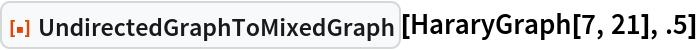Out=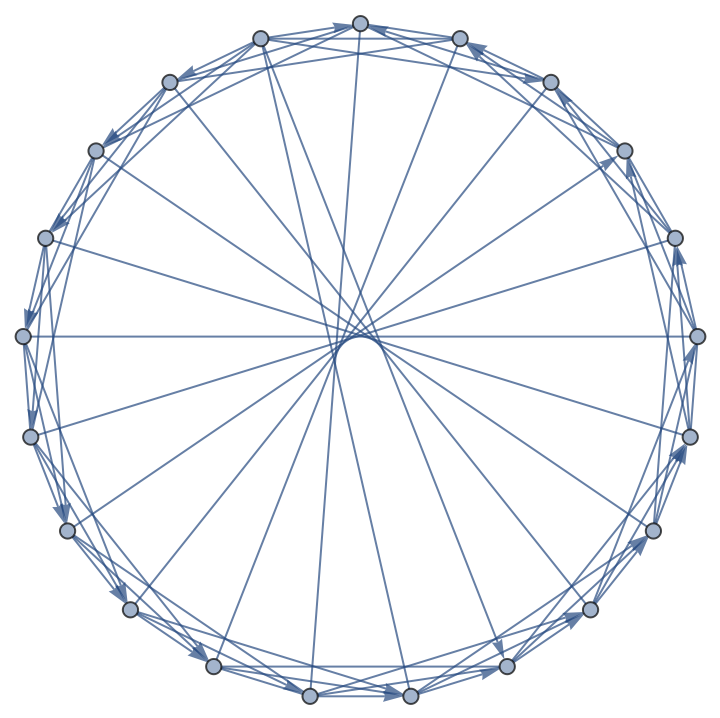Construct a circulant graph with 35% directed edges:

 In:=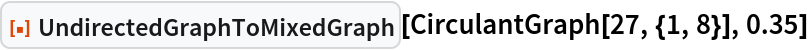Out=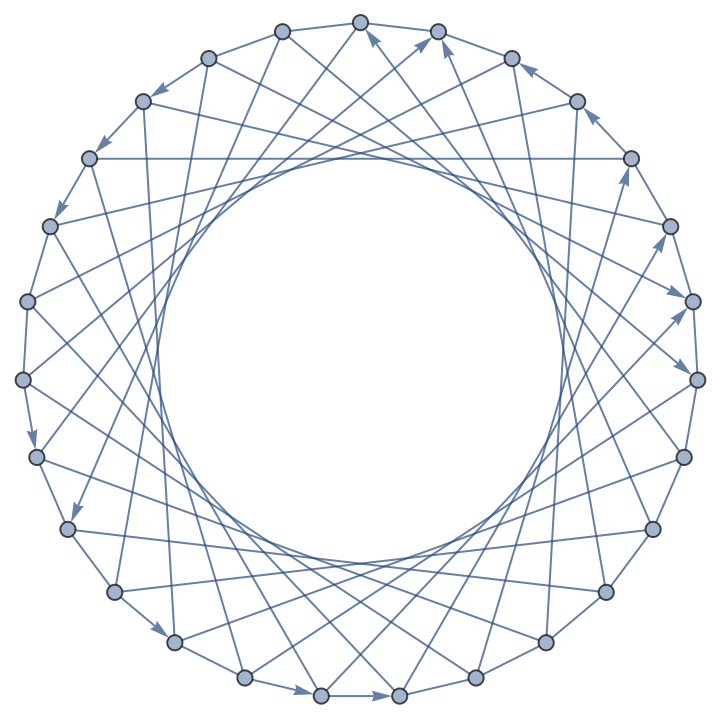Generate a random spatial graph and take the largest connected component by edge count:

 In:=In:=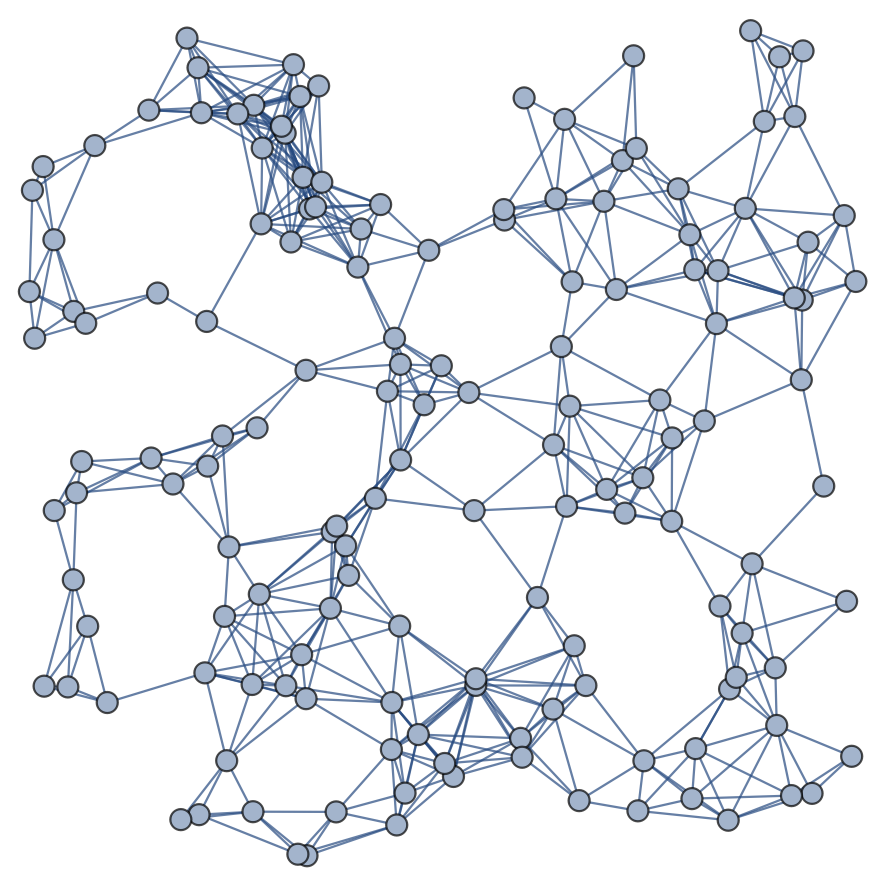Make the graph mixed with 50% directed edges:

 In:=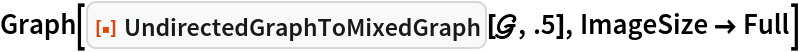In:=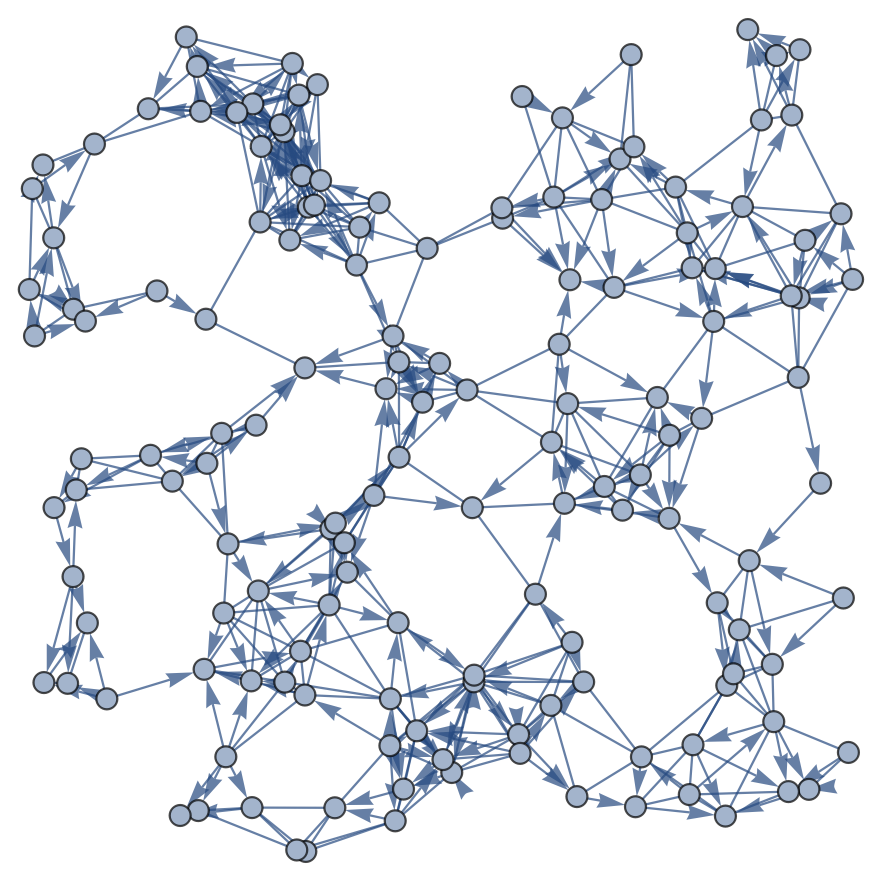### Applications (2)

Create a mixed dodecahedral graph with about 50% directed edges:

 In:=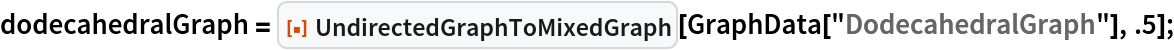Find a Hamiltonian cycle if it exists:

 In:=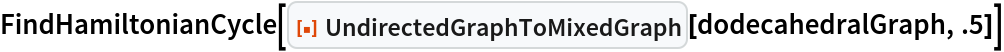Out=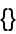Peter Burbery

## Version History

• 1.0.0 – 23 June 2022